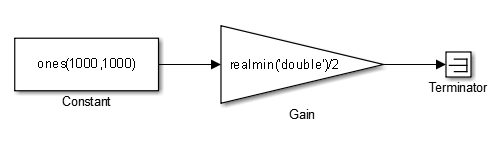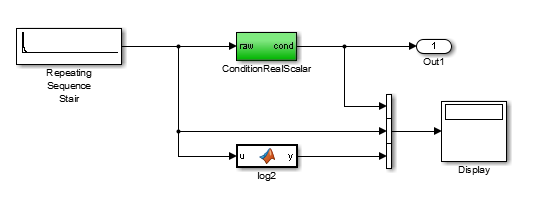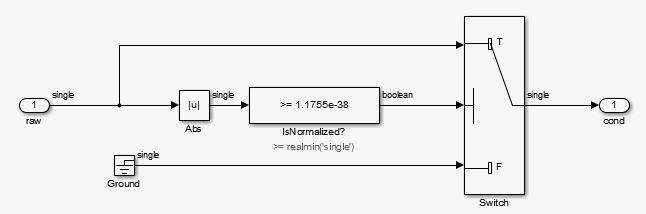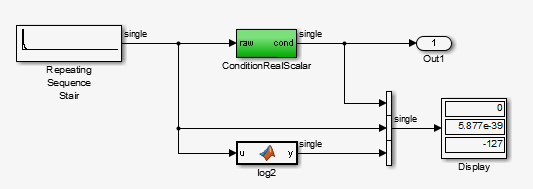Main Content

## Subnormal Number Execution Speed

Subnormal numbers, formerly known as denormal numbers in floating-point literature, fill the underflow gap around zero in floating-point arithmetic. Subnormal values are a special category of floating-point values that are too close to `0.0` to be represented as a normalized value. The leading significand (mantissa) of a subnormal number is zero. When adding and subtracting floating-point numbers, subnormal numbers prevent underflow.

Using subnormal numbers provides precision beyond the normal representation by using leading zeros in the significand to represent smaller values after the representation reaches the minimum exponent. As the value approaches `0.0`, you trade off precision for extended range. Subnormal numbers are useful if your application requires extra range.

However, in a real-time system, using subnormal numbers can dramatically increase execution latency, resulting in excessive design margins and real-time overruns. If the simulation or generated code performs calculations that produce or consume subnormal numbers, the execution of these calculations can be up to 50 times slower than similar calculations on normal numbers. The actual simulation or code execution time for subnormal number calculations depends on your computer operating environment. Typically, for desktop processors, the execution time for subnormal number calculations is five times slower than similar calculations on normal numbers.

To minimize the possibility of execution slowdowns or overruns due to subnormal number calculation latency, do one of the following:

• In your model, manually flush to zero any incoming or computed subnormal values at inputs and key operations, such as washouts and filters. For an example, see Flush Subnormal Numbers to Zero.

To detect a subnormal value for a single precision, 32-bit floating-point number:

1. Find the smallest normalized number on a MATLAB® host. In the Command Window, type:

`>> SmallestNormalSingle = realmin('single')`
In the C language, `FLT_MIN` , defined in `float.h`, is equivalent to``` realmin('single')```.

2. Look for values in range:

` 0 < fabsf(x) < SmallestNormalSingle `

To detect a subnormal value for a double precision, 64-bit floating-point number:

1. Find the smallest normalized number on a MATLAB host. In the Command Window, type:

`>> SmallestNormalDouble = realmin('double') `
In the C language, `DBL_MIN` , defined in `float.h`, is equivalent to``` realmin('double')```.

2. To detect a subnormal value, look for values in this range:

`0 < fabs(x) < SmallestNormalDouble`

• Set the Simulation behavior for denormal numbers parameter to ```Flush to zero (FTZ)``` to emulate flush-to-zero behavior for all denormal results from arithmetic operations. For more information, see Simulation behavior for denormal numbers.

• On your processor, set flush-to-zero mode or, with your compiler, specify an option to disable subnormal numbers. Flush-to-zero modes treat a subnormal number as 0 when it is an input to a floating-point operation. Underflow exceptions do not occur in flush-to-zero mode.

For example, in Intel® processors, the flush-to-zero (FTZ) and denormals-are-zero (DAZ) flags in the MXCSR register control floating-point calculations. For the gcc compiler on Linux, ` -ffast-math` sets abrupt underflow (FTZ), flush-to-zero, while ```–O3 -ffast-math``` reverts to gradual underflow, using subnormal numbers.

For more information, see the IEEE® Standard 754, IEEE Standard for Floating-Point Arithmetic.

### Simulation Time With and Without Subnormal Numbers

This model shows how using subnormal numbers increases simulation time by ~5 times.

Open the model ex_subnormal. The Gain is set to subnormal value `realmin('double')/2`.To run a simulation, in the Command Window, type ```for k=1:5, tic; sim('ex_subnormal'); toc,end```. Observe the elapsed times for simulation using subnormals, similar to the following:

```>> for k=1:5, tic; sim('ex_subnormal'); toc,end Elapsed time is 9.909326 seconds. Elapsed time is 9.617966 seconds. Elapsed time is 9.797183 seconds. Elapsed time is 9.702397 seconds. Elapsed time is 9.893946 seconds.```

Set the Gain to a number, `2`, that is not a subnormal value:

`>> set_param('ex_subnormal/Gain', 'Gain', '2');`

To run a simulation, in the Command Window, type ```for k=1:5, tic; sim('ex_subnormal'); toc,end```. Observe elapsed times for simulations that do not use subnormal values, similar to the following:

```>> for k=1:5, tic; sim('ex_subnormal'); toc,end Elapsed time is 2.045123 seconds. Elapsed time is 1.796598 seconds. Elapsed time is 1.758458 seconds. Elapsed time is 1.721721 seconds. Elapsed time is 1.780569 seconds.```

### Flush Subnormal Numbers to Zero

This example shows how to flush single precision subnormal numbers to zero.

1. Open the model ex_flush_to_zero:• `Repeating Sequence Stair` generates a sequence of numbers from two raised to the power of 0 through two raised to the power of -165. The sequence approaches zero.

• `ConditionRealScalar` flushes subnormal single precision values that are less than `realmin('single')` to zero.• MATLAB function block `log2` generates the base 2 logarithm of the ```Repeating Sequence Stair``` output. Specifically, `log2` generates the numbers 0 through -165.

2. On the Simulation > Step Back > Configure simulation stepping pane:

• Select Enable stepping back.

• Select Pause simulation when time reaches and enter `121`.

3. In the model window, run the simulation. The simulation pauses at `T=121`. The displayed values:

• `ConditionRealScalar` output approaches zero.

• ```Repeating Sequence Stair output``` approaches zero.

4. Step the simulation forward to `T=127`. `ConditionRealScalar` flushes the subnormal value output from ```Repeating Sequence Stair``` to zero.5. Continue stepping the simulation forward. `ConditionRealScalar` flushes the subnormal single precision values output from `Repeating Sequence Stair` to zero. When `T=150`, the output of `Repeating Squence Stair` is itself zero.

Download guide